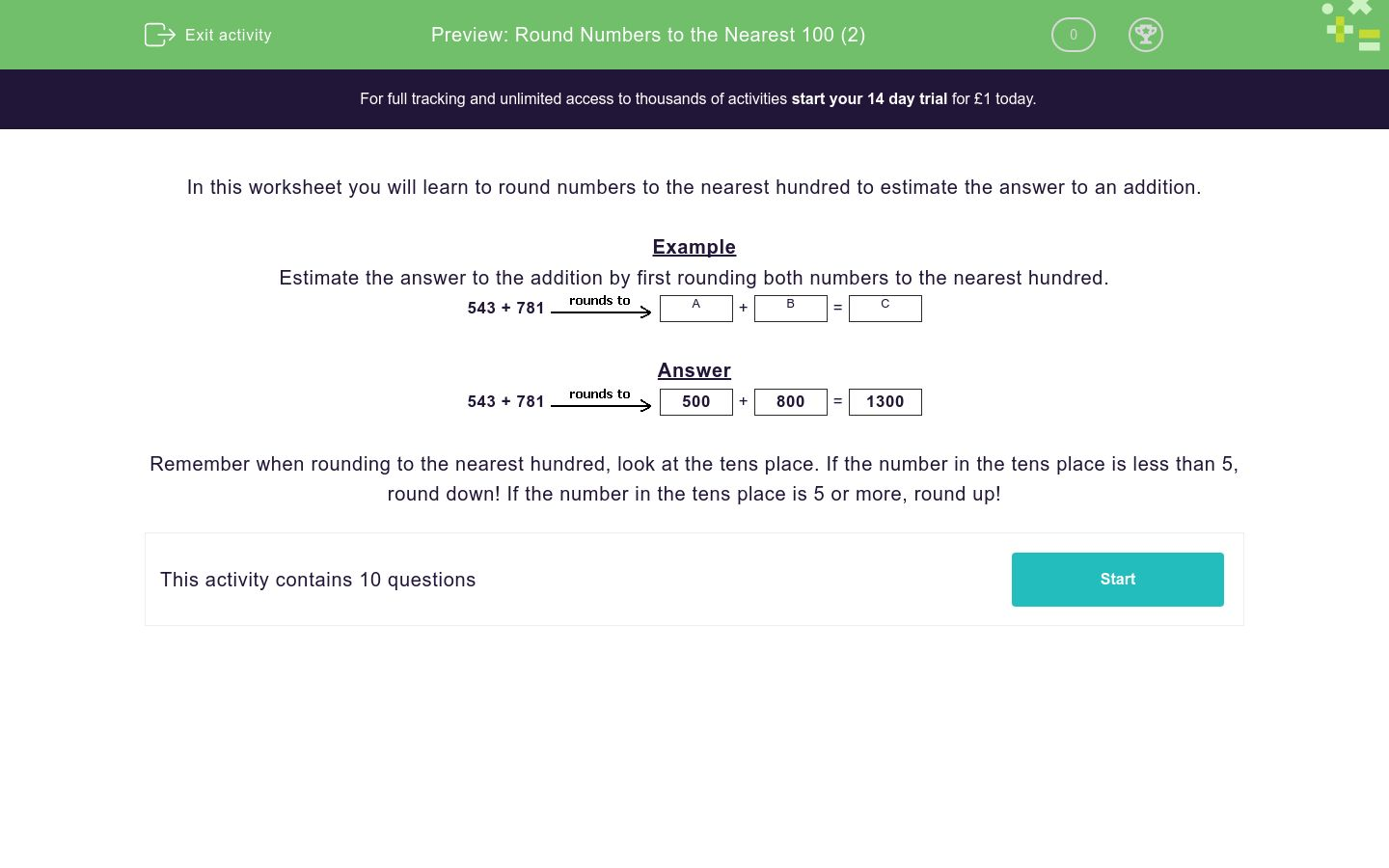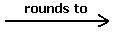# Round Numbers to the Nearest 100 (2)

In this worksheet, students learn to round numbers to the nearest hundred in order to estimate the answer to an addition.Key stage:  KS 2

Curriculum topic:   Number: Number and Place Value

Curriculum subtopic:   Round Numbers Up/Down (to 10, 100 or 1000)

Difficulty level:### QUESTION 1 of 10

In this worksheet you will learn to round numbers to the nearest hundred to estimate the answer to an addition.

Example

Estimate the answer to the addition by first rounding both numbers to the nearest hundred.

543 + 781A

+

 B

=

 C

543 + 781500

+

 800

=

 1300

Remember when rounding to the nearest hundred, look at the tens place. If the number in the tens place is less than 5, round down! If the number in the tens place is 5 or more, round up!

Estimate the answer by rounding the numbers to the nearest 100 before adding to find C.

685 + 425A
+
 B
=
 C

## Column B

A
700
B
400
C
1100

Estimate the answer by rounding the numbers to the nearest 100 before adding to find C.

717 + 225A
+
 B
=
 C

## Column B

A
900
B
700
C
200

Estimate the answer by rounding the numbers to the nearest 100 before adding to find C.

343 + 189A
+
 B
=
 C

## Column B

A
200
B
500
C
300

Estimate the answer by rounding the numbers to the nearest 100 before adding to find C.

112 + 124A
+
 B
=
 C

## Column B

A
200
B
100
C
100

Estimate the answer by rounding the numbers to the nearest 100 before adding to find C.

530 + 509A
+
 B
=
 C

## Column B

A
1000
B
500
C
500

Estimate the answer by rounding the numbers to the nearest 100 before adding to find C.

324 + 183A
+
 B
=
 C

## Column B

A
300
B
200
C
500

Estimate the answer by rounding the numbers to the nearest 100 before adding to find C.

314 + 93A
+
 B
=
 C

## Column B

A
100
B
400
C
300

Estimate the answer by rounding the numbers to the nearest 100 before adding to find C.

734 + 293A
+
 B
=
 C

## Column B

A
1000
B
300
C
700

Estimate the answer by rounding the numbers to the nearest 100 before adding to find C.

844 + 453A
+
 B
=
 C

## Column B

A
1300
B
500
C
800

Estimate the answer by rounding the numbers to the nearest 100 before adding to find C.

249 + 150A
+
 B
=
 C

## Column B

A
400
B
200
C
200
• Question 1

Estimate the answer by rounding the numbers to the nearest 100 before adding to find C.

685 + 425A
+
 B
=
 C

## Column B

A
700
B
400
C
1100
EDDIE SAYS
685 - The 80 in the tens place is more than 50, so round up to 700. 425 - The 20 in the tens place is less than 50, so round down to 400. 700 + 400 = 1100.
• Question 2

Estimate the answer by rounding the numbers to the nearest 100 before adding to find C.

717 + 225A
+
 B
=
 C

## Column B

A
700
B
200
C
900
EDDIE SAYS
717 - The 10 in the tens place is less than 50, so round down to 700. 225 - The 20 in the tens place is less than 50, so round down to 200. 700 + 200 = 900.
• Question 3

Estimate the answer by rounding the numbers to the nearest 100 before adding to find C.

343 + 189A
+
 B
=
 C

## Column B

A
300
B
200
C
500
EDDIE SAYS
343 - The 40 in the tens place is less than 50, so round down to 300. 189 - The 80 in the tens place is more than 50, so round up to 200. 300 + 200 = 500.
• Question 4

Estimate the answer by rounding the numbers to the nearest 100 before adding to find C.

112 + 124A
+
 B
=
 C

## Column B

A
100
B
100
C
200
EDDIE SAYS
112 - The 10 in the tens place is less than 50, so round down to 100. 124 - The 20 in the tens place is less than 50, so round down to 100. 100 + 100 = 200.
• Question 5

Estimate the answer by rounding the numbers to the nearest 100 before adding to find C.

530 + 509A
+
 B
=
 C

## Column B

A
500
B
500
C
1000
EDDIE SAYS
530 - The 30 in the tens place is less than 50, so round down to 500. 509 - The 0 in the tens place is less than 50, so round down to 500. 500 + 500 = 1000.
• Question 6

Estimate the answer by rounding the numbers to the nearest 100 before adding to find C.

324 + 183A
+
 B
=
 C

## Column B

A
300
B
200
C
500
EDDIE SAYS
324 - The 20 in the tens place is less than 50, so round down to 300. 183 - The 80 in the tens place is more than 50, so round up to 200. 300 + 200 = 500.
• Question 7

Estimate the answer by rounding the numbers to the nearest 100 before adding to find C.

314 + 93A
+
 B
=
 C

## Column B

A
300
B
100
C
400
EDDIE SAYS
314 - The 10 in the tens place is less than 50, so round down to 300. 93 - The 9 in the tens place is more than 5, so round up to 100. 300 + 100 = 400.
• Question 8

Estimate the answer by rounding the numbers to the nearest 100 before adding to find C.

734 + 293A
+
 B
=
 C

## Column B

A
700
B
300
C
1000
EDDIE SAYS
734 - The 30 in the tens place is less than 50, so round down to 700. 293 - The 90 in the tens place is more than 50, so round up to 300. 700 + 300 = 1000.
• Question 9

Estimate the answer by rounding the numbers to the nearest 100 before adding to find C.

844 + 453A
+
 B
=
 C

## Column B

A
800
B
500
C
1300
EDDIE SAYS
844 - The 40 in the tens place is less than 50, so round down to 800. 453 - There is a 50 in the tens place, so round up to 500. 800 + 500 = 1300.
• Question 10

Estimate the answer by rounding the numbers to the nearest 100 before adding to find C.

249 + 150A
+
 B
=
 C

## Column B

A
200
B
200
C
400
EDDIE SAYS
249 - The 40 in the tens place is less than 50, so round down to 200. 150 - There is a 50 in the tens place, so round up to 200. 200 + 200 = 400.
---- OR ----

Sign up for a £1 trial so you can track and measure your child's progress on this activity.

### What is EdPlace?

We're your National Curriculum aligned online education content provider helping each child succeed in English, maths and science from year 1 to GCSE. With an EdPlace account you’ll be able to track and measure progress, helping each child achieve their best. We build confidence and attainment by personalising each child’s learning at a level that suits them.

Get started